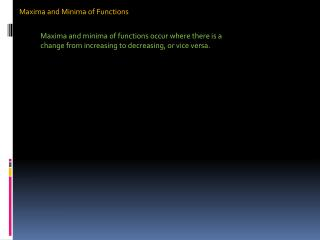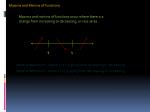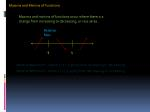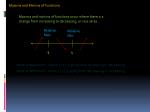Download PresentationMaxima and Minima of Functions

# Maxima and Minima of Functions - PowerPoint PPT Presentation

Maxima and Minima of Functions. Maxima and minima of functions occur where there is a change from increasing to decreasing, or vice versa. Maxima and Minima of Functions. Maxima and minima of functions occur where there is a change from increasing to decreasing, or vice versa. 4. -3.I am the owner, or an agent authorized to act on behalf of the owner, of the copyrighted work described.
Download Presentation## Maxima and Minima of Functions

An Image/Link below is provided (as is) to download presentation

Download Policy: Content on the Website is provided to you AS IS for your information and personal use and may not be sold / licensed / shared on other websites without getting consent from its author.While downloading, if for some reason you are not able to download a presentation, the publisher may have deleted the file from their server.

- - - - - - - - - - - - - - - - - - - - - - - - - - E N D - - - - - - - - - - - - - - - - - - - - - - - - - -
Presentation TranscriptMaxima and Minima of Functions

Maxima and minima of functions occur where there is a change from increasing to decreasing, or vice versa.Maxima and Minima of Functions

Maxima and minima of functions occur where there is a change from increasing to decreasing, or vice versa.

4

-3Maxima and Minima of Functions

Maxima and minima of functions occur where there is a change from increasing to decreasing, or vice versa.

Relative Max.

4

-3Maxima and Minima of Functions

Maxima and minima of functions occur where there is a change from increasing to decreasing, or vice versa.

Relative Max.

Relative Min.

4

-3Maxima and Minima of Functions

DECREASING

INCREASINGMaxima and Minima of Functions

INCREASING

DECREASING

INCREASINGMaxima and Minima of Functions

Relative Maximum

Relative MinimumMaxima and Minima of Functions

The difference in this example is we are restricted to a specific interval. So the edges of the interval will act as critical points along with the ones we find using the first derivative. They will be relative max or min depending on their position.Maxima and Minima of Functions

The difference in this example is we are restricted to a specific interval. So the edges of the interval will act as critical points along with the ones we find using the first derivative. They will be relative max or min depending on their position.

Moving left to right :

If the edge has a decreasing arrow following, it is a relative maximum.

If the edge has an increasing arrow following, it is a relative minimum.

If the edge has a decreasing arrow in front of it, it is a relative minimum.

If the edge has an increasing arrow in front of it, it is a relative maximum.Maxima and Minima of Functions

Moving left to right :

If the edge has a decreasing arrow following, it is a relative maximum.

If the edge has an increasing arrow following, it is a relative minimum.

If the edge has a decreasing arrow in front of it, it is a relative minimum.

If the edge has an increasing arrow in front of it, it is a relative maximum.Maxima and Minima of Functions

Moving left to right :

If the edge has a decreasing arrow following, it is a relative maximum.

If the edge has an increasing arrow following, it is a relative minimum.

If the edge has a decreasing arrow in front of it, it is a relative minimum.

If the edge has an increasing arrow in front of it, it is a relative maximum.Maxima and Minima of Functions

Moving left to right :

If the edge has a decreasing arrow following, it is a relative maximum.

If the edge has an increasing arrow following, it is a relative minimum.

If the edge has a decreasing arrow in front of it, it is a relative minimum.

If the edge has an increasing arrow in front of it, it is a relative maximum.Maxima and Minima of Functions

Moving left to right :

If the edge has a decreasing arrow following, it is a relative maximum.

If the edge has an increasing arrow following, it is a relative minimum.

If the edge has a decreasing arrow in front of it, it is a relative minimum.

If the edge has an increasing arrow in front of it, it is a relative maximum.Maxima and Minima of Functions

Moving left to right :

If the edge has a decreasing arrow following, it is a relative maximum.

If the edge has an increasing arrow following, it is a relative minimum.

If the edge has a decreasing arrow in front of it, it is a relative minimum.

If the edge has an increasing arrow in front of it, it is a relative maximum.Maxima and Minima of Functions

Moving left to right :

If the edge has a decreasing arrow following, it is a relative maximum.

If the edge has an increasing arrow following, it is a relative minimum.

If the edge has a decreasing arrow in front of it, it is a relative minimum.

If the edge has an increasing arrow in front of it, it is a relative maximum.Maxima and Minima of Functions

Moving left to right :

If the edge has a decreasing arrow following, it is a relative maximum.

If the edge has an increasing arrow following, it is a relative minimum.

If the edge has a decreasing arrow in front of it, it is a relative minimum.

If the edge has an increasing arrow in front of it, it is a relative maximum.Maxima and Minima of Functions

Relative Maximum

Moving left to right :

If the edge has a decreasing arrow following, it is a relative maximum.

If the edge has an increasing arrow following, it is a relative minimum.

If the edge has a decreasing arrow in front of it, it is a relative minimum.

If the edge has an increasing arrow in front of it, it is a relative maximum.Maxima and Minima of Functions

Relative Maximum

This is neither because there is no change in increasing/decreasing. It is called an “inflection point” which we will discuss later…

Moving left to right :

If the edge has a decreasing arrow following, it is a relative maximum.

If the edge has an increasing arrow following, it is a relative minimum.

If the edge has a decreasing arrow in front of it, it is a relative minimum.

If the edge has an increasing arrow in front of it, it is a relative maximum.Maxima and Minima of Functions

Relative Maximum

Relative Minimum

Change from decreasing to increasing…

Moving left to right :

If the edge has a decreasing arrow following, it is a relative maximum.

If the edge has an increasing arrow following, it is a relative minimum.

If the edge has a decreasing arrow in front of it, it is a relative minimum.

If the edge has an increasing arrow in front of it, it is a relative maximum.Maxima and Minima of Functions

Relative Maximum

Relative Maximum

Relative Minimum

Moving left to right :

If the edge has a decreasing arrow following, it is a relative maximum.

If the edge has an increasing arrow following, it is a relative minimum.

If the edge has a decreasing arrow in front of it, it is a relative minimum.

If the edge has an increasing arrow in front of it, it is a relative maximum.